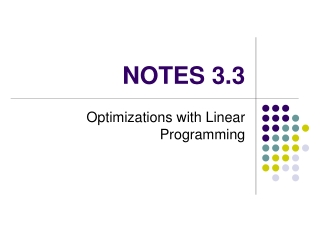DownloadDownload PresentationNOTES 3.3

# NOTES 3.3

Télécharger la présentation## NOTES 3.3

- - - - - - - - - - - - - - - - - - - - - - - - - - - E N D - - - - - - - - - - - - - - - - - - - - - - - - - - -
##### Presentation Transcript

1. NOTES 3.3 Optimizations with Linear Programming

2. Feasible Region: • Linear Programming: used to find the maximum and minimum values of a system of inequalities Constraint: • the restriction contained in the problem • the solution (shaded region) to the set of constraints

3. Objective Function: the function to be minimized or maximized (in the form: C = Ax + By) • Optimization – finding the maximum or minimum value of some quantity.

4. Step 1: Determine variables, objective function, and constraints Step 2: Graph the constraints. Step 3: Find the vertices (the ordered pairs that are corner points) Step 4: Test the vertices in the objective function bounded region – find both max and min unbounded region – find either max or min, but not both pick a point in the solution set and eliminate the “min” or “max” Step 5: Answer the question

5. EX 1: Constraints x > 2 x < 5 y > 0 y < –2x + 12 Optimize the Objective Function: C = –x + 3y

6. EX 2: Objective Function: C = x + 5y Constraints: x > 0 y < 2x + 2 5 > x +y

7. EX 3: Objective Function: C = 2x – y Restraints: x > 0 y ≤x + 2 y ≤ –x +6

8. EX 4. A T-shirt Company makes long & short sleeve t-shirts. It costs \$4 to make the short sleeve t-shirts and it costs \$6 to make the long sleeve t-shirts. Production costs cannot exceed \$4800 and the machines cannot make more than 1000 t-shirts a day. The profit for making short sleeve shirts is \$2.50 per shirt while the company makes \$4.50 for each long sleeve shirt. What are the maximum and minimum profits the company can make? (a) WRITE A SYSTEM OF INEQUALITIES TO REPRESENT THE CONSTRAINTS, Let x = _____________________ Let y = ________________________ (b) GRAPH THE FEASIBLE REGION, (c) WRITE AN OBJECTIVE FUNCTION FOR THE PROFIT P = (d) MAXIMIZE THE OBJECTIVE FUNCTION

9. EX 5: Nicole's Nutrition Center sells health food to mountain-climbing teams. The Trailblazer mix package contains one pound of corn cereal mixed with four pounds of wheat cereal and sells for \$9.75. The Frontier mix contains two pounds of corn cereal mixed with three pounds of wheat cereal and sells for \$9.50. The center has available 60 pounds of corn cereal and 120 pounds of wheat cereal. How many packages of each mix should the center sell to maximize its income? Let x = _____________________ Let y = ____________________ (a) Write a system of inequalities to represent the constraints. (b) Graph the feasible region (c ) Write an objective function for the company’s total profit, P. P = (d) Maximize the objective function.